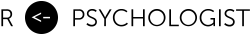# Estimating treatment effects and ICCs from (G)LMMs on the observed scale using Bayes, Part 1: lognormal models

August 05, 2018

When a multilevel model includes either a non-linear transformation (such as the log-transformation) of the response variable, or of the expectations via a GLM link-function, then the interpretation of the results will be different compared to a standard Gaussian multilevel model; specifically, the estimates will be on a transformed scale and not in the original units, and the effects will no longer refer to the average effect in the population, instead they are conditional/cluster-specific. In this post, I will deal with linear mixed-effects models (LMM) that use a log-transformed outcome variable. This will be the first part of a three-part tutorial on some of the finer details of (G)LMMs, and how Bayes can make your (frequentist) life easier. In each post I will focus on:

• log-transformation of the dependent variable in a multilevel model
• Calculating both multiplicative effects (% change) and differences on the untransformed scale.
• Subject-specific/cluster-specific versus population-average effects (conditional versus marginal effects).
• Intraclass correlations on the transformed/link/latent scale or on the response/data/original scale.

In part 2 I will cover a GLMM with a binary outcome, and part 3 will focus on semicontinuous (hurdle/two-part) models when the outcome is a skewed continuous variable that include zeros.

The aim of this post is also to show:

• How simulation-based approaches like MCMC make it much easier to make inferences about transformed parameters.
• How the estimates from a multilevel model can be transformed to answer the same questions as population-average models or fixed effects models.

## Data-generating model: a lognormal LMM

As an example, we will use a simple hierarchical design with clusters nested within either a treatment or a control condition. This is a classic 2-level multilevel model.

In this model, the natural logarithm of is normally distributed conditional on the cluster-specific effect and the treatment variable . Let’s assume is the average money (in USD) lost gambling per week (since I do research on gambling disorder). Gambling expenditure is sometimes quite well described by a lognormal distribution, or a (generalized) Gamma distribution. Importantly, the methods in this post can quite easily be tweaked to work with other response distributions. Each treatment arm has clusters, and each cluster has observations. In this example clusters refer to e.g. schools, centers, groups, and the first level are subjects. However, it would be easy to use the contents of this post for a longitudinal model where clusters refer to subjects, and the subjects have repeated observations.

## Simulate data

Let’s create a simple function that generates data from this model

The model parameters are

## Fit the model

We can fit this model using brms (or rstanarm, lme4, etc).

Using the notation of our 2-level model, we get these results

ParameterEstimate
6.21
-0.70
0.64
0.51

## Interpreting the parameters

All of the estimates are on the log scale, and they do not have an immediate interpretation on the natural scale. To further complicate things we should also remind ourselves that the effects from (G)LMM have cluster-specific interpretations (“subject-specific” in a longitudinal model when clusters = subjects). Researchers who work mostly with LMMs sometimes tend to forget this, since for LMMs the cluster-specific effects are equal to the population-average (marginal) effects. The non-linear transformation of either the data (like the log-transformation) or via the link function in (G)LMMs both means that back-transformation of the model’s estimates from e.g. the log scale does not give the expected values on the natural scale (cf. Jensen’s inequality).

In the figure below I try to illustrate both the cluster-specific nature of the model, and the transformation between the log scale and the natural data scale, without being too technical.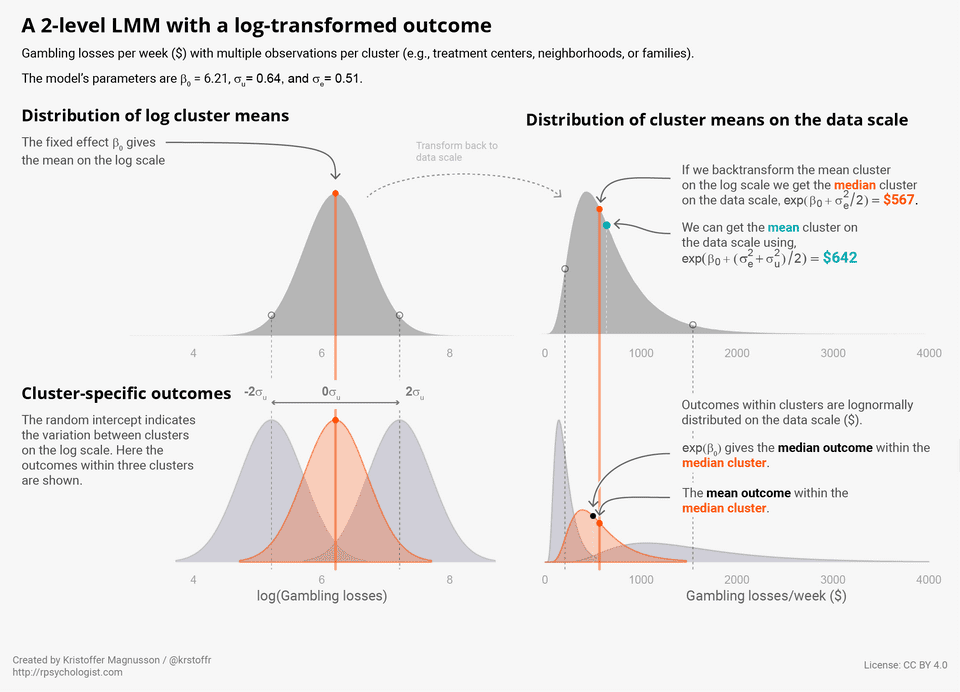### How to calculate marginal effects on the data scale

So how do we derive the numbers presented in the figure above? Well, for the lognormal distribution with the log mean and the log SD we have:

• mean:
• median:

If we want to get the marginal mean we need to average this back-transformation over the random effects distribution. Since, as noted earlier, simply back-transforming the log coefficient (the expected mean on the log scale) will not give the expected mean on the original scale (money lost).

The correct expectation is

This integral is easily solved using numerical integration.

In this case, we can also derive an exact and simple solution using the moment-generating function of the lognormal distribution (see Proof 1: left as an exercise to the reader 🤓)

A third option is Monte Carlo integration. Were we simply simulate a large number of cluster outcomes on the log scale, back-transform to the natural scale, and then get the average. For multivariate integrals (e.g. models with correlated random effects) this is often the simplest solution that also works well in practice, although the package cubature offers a fast solution using adaptive numerical integration.

We see that all three methods give the same result (within Monte Carlo error).

### Can we interpret the cluster-specific values?

So, if the expected value for the outcome group is 642 USD, and = 567 USD is there a meaningful interpretation of 567? As shown in the figure above, this is the expected value for the cluster at the center of the distribution of cluster means on the log scale. Since percentiles are invariant under a monotonic transformation (and the mean is equal to the median for the normal distribution), this cluster (with ) is also at the median on the natural scale.

So to summarize, the cluster-specific effects are,

and the expected marginal mean in each treatment group is,

### Average treatment effects on the data scale

Using the results above, the cluster-specific average treatment effect on the original scale is,

which is (for ),

And the marginal treatment effect on the original scale is,

### Multiplicate effects (% change)

Most know that if we take the exponent of the log difference we get the % change. Generally, these multiplicative effects are also cluster-specific. However, for our simple model gives both the cluster-specific and population-average effect. We can see this if we look at the ratio of the expected conditional outcome in the treatment and control group,

and similarly for the marginal outcomes

The cluster-specific and population-average effect is the same. However, this is not true in general for nonlinear transformation or GLMM link functions (which we shall see in part 2 on binary outcomes).

### 95 % CIs for the backtransformed marginal effects

Getting back to our fitted model, let’s see how we can transform the estimates into the posterior distribution of e.g. the marginal mean outcome in the control group. We do this by applying the back-transformation and marginalization using all the posterior samples of , , and .

As one would expect, estimates of the population effect are less precise compared to condition on a specific cluster effect.

### Summarizing the model

Let’s summarize all the results from the model, both the conditional and marginal, absolute and multiplicative treatment effects.

EffectFormulaposterior_medianl_95_CIu_95_CI
Average outcome for a median cluster in the control group570.5374.5847.9
Average outcome for a median cluster in the treatment group281.8186.7435.2
Average outcome in the control group697.9454.51123.4
Average outcome in the treatment group342.7231.3575.5
Difference between the average outcome in a median cluster in the treatment versus control group-286.1-580.2-37.9
Difference between the average outcome in the treatment versus control group-349.0-747.5-47.4
Ratio of the average outcome in a median cluster in the treatment and the control group0.50.30.9
Ratio of the average outcome in the treatment and the control group0.50.30.9
Ratio of the average outcome in the treatment and the control group0.50.30.9

### Using brms::fitted

Let’s compare our results to the predicted means we get with brms::fitted and brms::marginal_effects

We see that this is exactly the same as the median outcome (cluster-specific outcome) in the control group. I’ve seen some people confused about this since the function is called marginal_effects.

### Limiting inference to only the included clusters

We can also use fitted to average over the included clusters, this is very similar to fitting the clusters as “fixed effects”. So let’s compare averaging over the included clusters in the multilevel model to fitting a fixed effects (no pooling) model.

Let’s look at the predicted mean for the control group using the fixed effects model.

Which is really similar to the control groups sample mean which is 636. Now let’s compare the posterior distribution from the fixed effects model, to using our multilevel model to average over only the included clusters (and not the estimated distribution of clusters).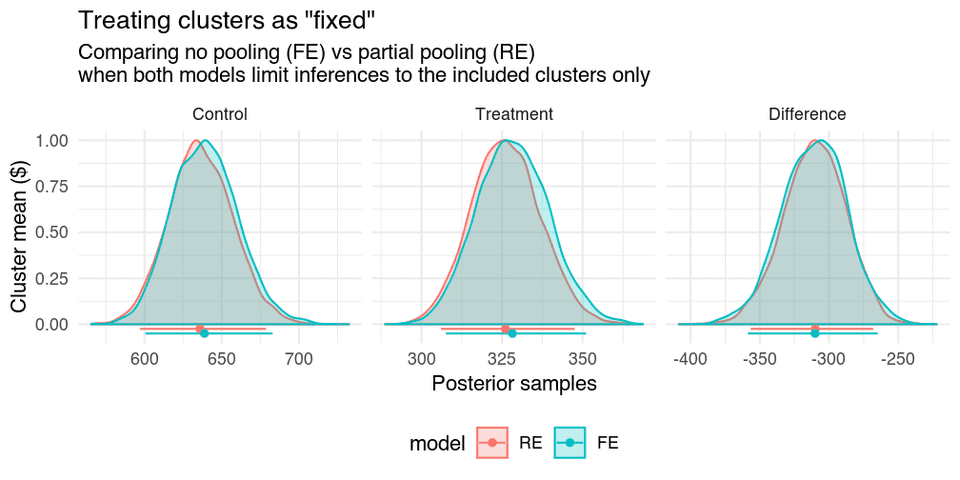We see that the results are highly similar. Although, the models behind the results do differ. If we look at the estimates for the specific clusters we can see the—in this model very weak—effect of partial pooling in the multilevel model.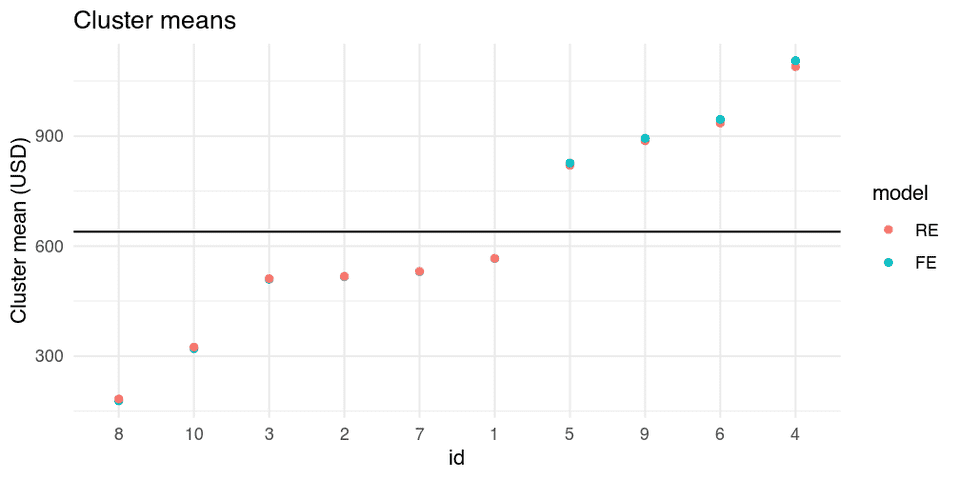## Intraclass correlations (ICC)

Since the variance components are on the log scale ICCs calculated using them will also be on the log scale. This is not always what we want, for instance, if we are calculating agreement, reliability, or heritability, it can make a lot of sense to calculate ICCs on the data scale. If I am calculating ICCs for family members’ reports on gambling losses, then I am interested in agreement on the data scale (USD) and not on the log scale.

ICCs on the data scale can be calculated using the same techniques we used for the marginalization of the outcomes. You can read more about this in the context of GLMMs in Villemereuil et al. (2016; and the related R package QQglmm). Here is an example, first we calculate the marginal mean

then we use the marginal mean to calculate the expected variance between clusters on the data scale,

and lastly we calculate the expected variance within clusters on the data scale,

Where is the variance of the lognormal distribution evaluated at .

The ICC on the response scale in then

Whereas, the log scale ICC is,

Since this is also a one-dimensional integral we can use integrate,

If you find simulations more intuitive, the Monte Carlo version would be

Let’s see if the two methods agree.

Data scale (Integrate)Data scale (Simulate)Log scale
ICC0.440.440.5
WI_sd387.71387.69NA
BW_sd342.15342.22NA
mean642.01641.93NA

Great! We also see that by calculating variances on the data scale the ICCs are lower, in this case, the difference is small, but if the log variance is large this difference can be very large. So when you see ICCs from lognormal models or GLMMs you should ask if they are on the transformed (latent) scale or on the observed (data) scale, and if the scale is appropriate for the research question.

## Simulation study

I began by promising that the methods in this post could make your frequentist life easier, and then continued to only fit Bayesian models. So let’s do a small simulation study to evaluate the frequentist properties of this model and all the transformations and marginalizations. I will try two different models, shown in the table below, each model will be evaluated using 5000 replications, and I will fit both the correct model using a lognormal response distribution and a standard LMM using the Gaussian response distribution.

ParameterSim1: less skewSim2: more skew
log(500)log(500)
log(0.5)log(0.5)
0.51
0.51
305
1075

### Simulation results

In the figures below we see that the posterior median show mostly negligible bias for both the original parameters and the transformed marginal effects. The Gaussian model has the most trouble with the variance components, which show substantial bias.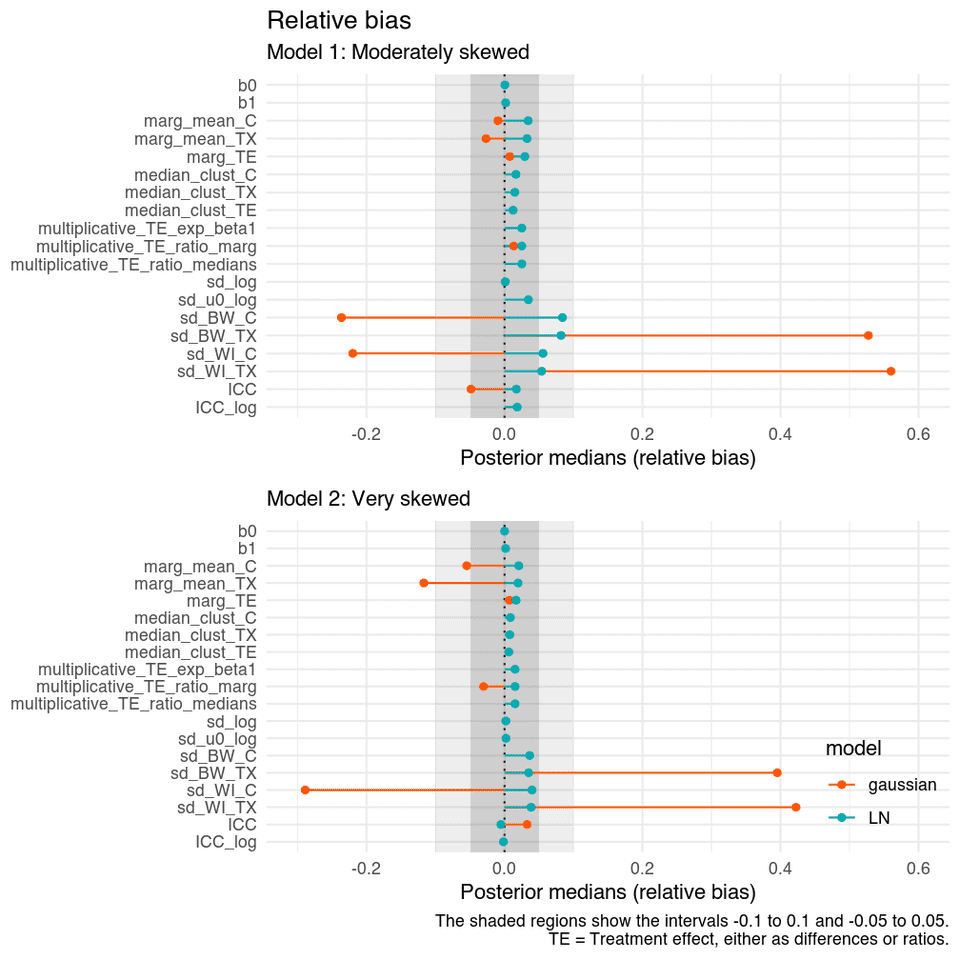If we look at the coverage probabilities we see that the lognormal model’s posterior intervals have nominal coverage rates for all parameters. The Gaussian model has slightly worse coverage for the treatment effects, but not that bad. However, the CI’s from the Gaussian model does a poor job of capturing the true ICC and variance components.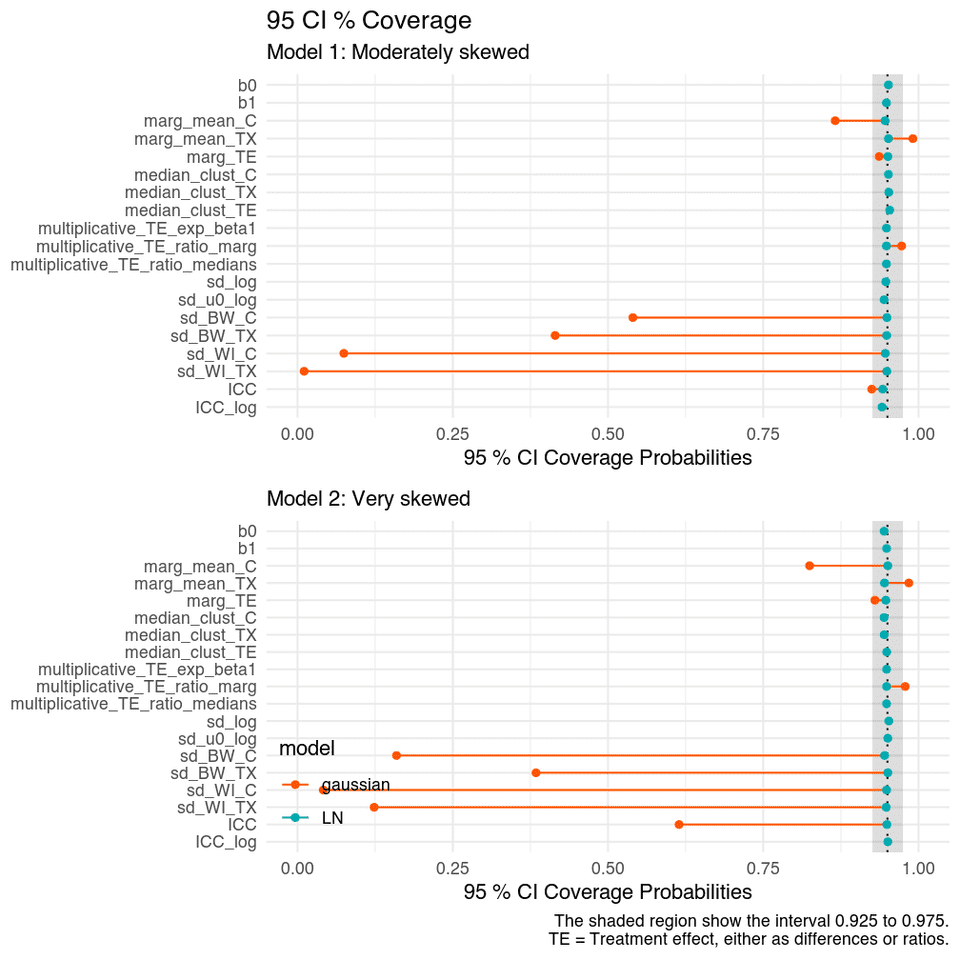### Power

Lastly, let’s take a closer look at the marginalized treatment effect on the data scale. The figure below shows a sample of the confidence intervals for the difference in the average money lost gambling from the two conditions, from Model 2 with more skewed data. We can see that the, although, the normal model is quite robust and the estimated effect is unbiased, it is less precise which leads to reduced power and CIs with worse coverage compared to the true lognormal model.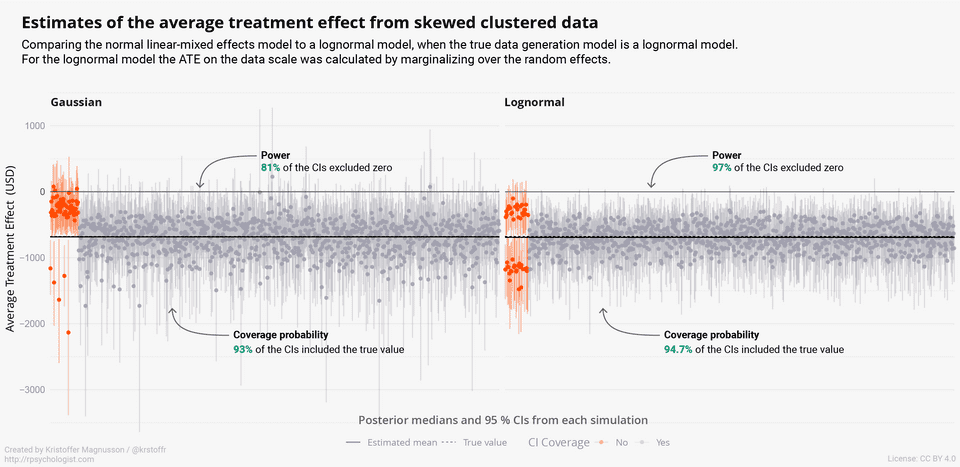## Summary

There is a lot of information in this post, so let’s summarize the key take-home messages:

• Both cluster-specific (conditional) and population-average (marginal) treatment effects are useful—they just answer different questions and we should be careful to not mixed them up.
• The multilevel model can be used to give both cluster-specific (conditional) and population-average effects on both the original and transformed scale while retaining all of the advantages of likelihood-based/Bayesian methods.
• There is no free lunch. We saw that the calculations of the marginal effects on the data scale use estimates of both the lognormal variance, as well as the random intercept. Thus, model misspecification will have a large impact on the appropriateness of the back-transformation. In that sense, directly modeling the population-average effects using e.g. GEE and applying nonparametric retransformations (e.g. smearing) is more robust in some aspects. However, once we add complexities like MNAR missing data in combination with weak adherence (both by patients and therapists…) to complex interventions, then it can be argued that a carefully specified generative model that combines both statistical and clinical expertise will be more likely to give useful answers regarding the treatment effect. In my experience, such models will often have multilevel and cluster-specific components.
• For outcomes that are highly meaningful on the original scale (such as expenditures), absolute effects on the data scale can be very meaningful.
• Using the default priors in brms resulted in frequentist CI’s with nominal coverage probabilities.
• It is useful to understand how the conditional and marginal effects relate to each other, to avoid misinterpretations of the default cluster-specific effects (very common in the clinical studies I read).

The issues covered in this post is dealt with in many articles, here is a selection of relevant articles:

• Gromping, U. (1996). A Note on Fitting a Marginal Model to Mixed Effects Log-Linear Regression Data via GEE. Biometrics, 52(1), 280–285. https://doi.org/10.2307/2533162
• Heagerty, P. J., & Zeger, S. L. (2000). Marginalized multilevel models and likelihood inference (with comments and a rejoinder by the authors). Statistical Science, 15(1), 1–26. https://doi.org/10.1214/ss/1009212671
• Hedeker, D., Toit, S. H. C. du, Demirtas, H., & Gibbons, R. D. (2018). A note on marginalization of regression parameters from mixed models of binary outcomes. Biometrics, 74(1), 354–361. https://doi.org/10.1111/biom.12707
• Villemereuil, P. de, Schielzeth, H., Nakagawa, S., & Morrissey, M. (2016). General Methods for Evolutionary Quantitative Genetic Inference from Generalized Mixed Models. Genetics, 204(3), 1281–1294. https://doi.org/10.1534/genetics.115.186536

## Simulation code

run_sim.R: perform the simulation

summarise_sim.R: Summarize results

functions.R: Functions used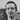Written by Kristoffer Magnusson a researcher in clinical psychology. You should follow him on Twitter and come hang out on the open science discord Git Gud Science.

Share:

Published August 05, 2018 (View on GitHub)